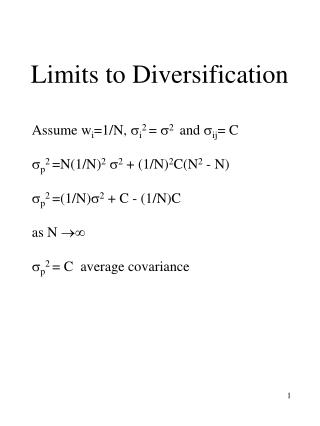# Limits to Diversification - PowerPoint PPT PresentationDownload PresentationLimits to Diversification

Limits to DiversificationDownload Presentation## Limits to Diversification

- - - - - - - - - - - - - - - - - - - - - - - - - - - E N D - - - - - - - - - - - - - - - - - - - - - - - - - - -
##### Presentation Transcript

1. Limits to Diversification Assume wi=1/N, i2 = 2 and ij= C p2 =N(1/N)2 2 + (1/N)2C(N2 - N) p2 =(1/N)2 + C - (1/N)C as N  p2 = C average covariance

2. Presence of Risk free Security Rf = risk free rate 2f = 0 Combining risk free asset and a risky portfolio: E(Rp) = wf E(Rf) + wA E(RA) p2 =wA A2 E(RA) Rf A

3. Efficient Frontier E(R) Efficient frontier Opportunity set  Efficient Frontier: the upper boundary of the opportunity set

4. Assumptions • Investors can choose on the basis of mean-variance criterion • Normal distribution of asset returns or quadratic utility function • Investors have homogeneous expectations • planning horizon • distribution of security returns • There are no frictions in the capital markets • no transactions costs • no taxes on dividends, capital gains, interest income • Information available at no cost

5. Efficient Frontier with Risk Free Security E(R) M Rf  M is the market portfolio

6. Risk and Return Capital Market Line: Separation Theorem: The determination of optimal portfolio of risky assets is independent from individual’s risk preferences.

7. Contribution to portfolio risk The risk that an individual stock contributes to the risk of a portfolio depends on: -proportion invested in that stock, wi -its covariance with the portfolio, iM Therefore, Contribution to risk = wi iM Ratio of stock i’s contribution to the risk of portfolio: wi iM / 2M The ratio iM / 2M = beta coefficient

8. Capital Asset Pricing Model E(RM) - Rf = Risk premium on the market E(Ri) - Rf = Risk premium on stock i An investor can always obtain a risk premium BA(E(RM) - Rf) by combining M and the risk free asset. Thus: E(Ri) - Rf = Bi(E(RM) - Rf) or E(Ri) = Rf + Bi(E(RM) - Rf)

9. Security Market Line E(R) E(RM) Rf B BM=1